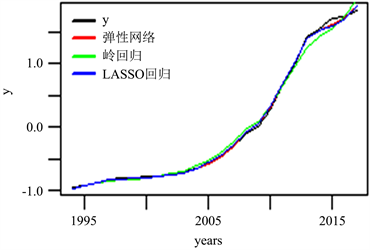# 基于弹性网回归的云南省财政收入影响因素分析Analysis on the Influencing Factors of Yunnan Province’s Fiscal Revenue Based on Elastic Net

DOI: 10.12677/SA.2021.103041, PDF, HTML, XML, 下载: 38  浏览: 90

Abstract: Economic variables often have strong correlations with each other, complicating the model. In this paper, we conduct a multiple collinearity test on the original data at first; then, Yunnan Province’s fiscal revenue related data are modeling and analyzed by cross validation method. Finally, the results of Elastic net regression and Ridge regression and LASSO regression estimations are analyzed and compared. At the same time, it is concluded that the Yunnan Province’s fiscal revenue is affected by tax revenue, regional gross domestic product, total retail sales of consumer goods, total wages of employed workers, number of social employment, output value of primary industry, investment in fixed assets of the whole society and total income of tourism province.

1. 引言

2. 弹性网回归

$\underset{\beta \in {R}^{p}}{\mathrm{arg}\mathrm{min}}\left\{{‖y-X\beta ‖}^{2}+\lambda \left[\left(1-\alpha \right){‖\beta ‖}_{2}+\alpha {‖\beta ‖}_{1}\right]\right\}$

3. 实证分析

3.1. 多重共线性检验

3.2. 模型比较与分析Table 2. Coefficient estimation for each regression method (retaining four decimal places)Table 3. RMSE, R square of three methodsFigure 1. Effect drawing of three methods

$\begin{array}{c}{y}^{*}=0.4978{X}_{1}^{*}+0.0703{X}_{2}^{*}+0.1576{X}_{3}^{*}+0.0497{X}_{4}^{*}-0.054{X}_{6}^{*}\\ \text{ }\text{\hspace{0.17em}}\text{\hspace{0.17em}}+0.2202{X}_{7}^{*}+0.1217{X}_{9}^{*}-0.066{X}_{10}^{*}\end{array}$

$\begin{array}{c}y=4298702819+144473.2{X}_{1}+250194.5{X}_{2}+248995.4{X}_{3}+24005.87{X}_{4}\\ \text{\hspace{0.17em}}\text{\hspace{0.17em}}-10717.22{X}_{6}+98663.57{X}_{7}+435045.2{X}_{9}-72750.11{X}_{10}\end{array}$

4. 结论

  朱德云, 李萌. 经济欠发达地区财政收入增长影响因素研究——基于山东菏泽的样本分析[J]. 财贸经济, 2012(7): 21-28.  毛琴, 李明江, 刘彦. 基于逐步回归法的国家财政收入数据回归模型分析[J]. 电子技术与软件工程, 2013(19): 227-228.  刘心竹, 李昊, 刘青青, 等. 我国地方财政收入影响因素的实证分析[J]. 中国集体经济, 2012(9): 84-85.  邓洁. 我国财政收入影响因素的实证分析[J]. 金卡工程, 2009, 13(9): 180-181.  Zou, H. and Hastie, T. (2005) Addendum: “Regularization and Variable Selection via the Elastic Net”. Journal of the Royal Statistical Society: Series B (Statistical Methodology), 67, 301-320. https://doi.org/10.1111/j.1467-9868.2005.00503.x  Hoerl, A.E. and Kennard, R.W. (1970) Ridge Regression: Applications to Nonorthogonal Problems. Technometrics, 12, 69-82. https://doi.org/10.1080/00401706.1970.10488635  Hoerl, A.E. and Kennard, R.W. (1970) Ridge Regression: Biased Estimation for Nonorthogonal Problems. Technometrics, 12, 55-67. https://doi.org/10.1080/00401706.1970.10488634  Tibshirani, R. (2011) Regression Shrinkage and Selection via the Lasso: A Retrospective. Journal of the Royal Statistical Society: Series B (Statistical Methodology), 73, 267-288. https://doi.org/10.1111/j.1467-9868.2011.00771.x  Zou, H. (2006) The Adaptive Lasso and Its Oracle Properties. Journal of the American Statistical Association, 101, 1418- 1429.